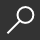## Real Estate Math Express : Rapid Review and Practice with Essential License Exam Calculations by David Cusic and Ryan Mettling and Stephen Mettling

Overview -

Real Estate Math Express is a concise, easy-to-study test preparation guide to help real estate students improve their real estate math scores to pass the state licensing test. The primary feature of Real Estate Math Express is that it contains all necessary formulas and practice questions in 70+ pages. This enables you to truly cram for the math portion of your state's licensure test, memorize key formulas, and walk into the exam site having all the essential material in your short-term memory

So, if you want a truly rapid review resource, Real Estate Math Express is for you. No frills, no lengthy explanations.....just key formulas, definitions, corresponding examples and practice questions.

Real Estate Math Express can be broken down into 3 major parts:

• Math Formulary
• Math Questions

• The math formulary is the section that introduces each major real estate math topic and its corresponding formula(s). Real Estate Math Express covers numerous topics ranging from basic math to capitalization rates to prorations and finally to closing cost calculations.

Once the math formulas have been mastered, there are over 110 sample questions for you to practice with. Each question has both an answer and an explanation of the correct answer at the end of the book.

For additional real estate licensing materials, please check out our Principles of Real Estate Practice and Real Estate License Exam Prep (RELEP) Series.

local_shippingFor DeliveryIn Stock.
This item is Non-Returnable.
FREE Shipping for Club Members helpNew & Used Marketplace 8 copies from \$6.96

## More About Real Estate Math Express by David Cusic; Ryan Mettling; Stephen Mettling

### Overview

Real Estate Math Express is a concise, easy-to-study test preparation guide to help real estate students improve their real estate math scores to pass the state licensing test. The primary feature of Real Estate Math Express is that it contains all necessary formulas and practice questions in 70+ pages. This enables you to truly cram for the math portion of your state's licensure test, memorize key formulas, and walk into the exam site having all the essential material in your short-term memory

So, if you want a truly rapid review resource, Real Estate Math Express is for you. No frills, no lengthy explanations.....just key formulas, definitions, corresponding examples and practice questions.

Real Estate Math Express can be broken down into 3 major parts:

• Math Formulary
• Math Questions

• The math formulary is the section that introduces each major real estate math topic and its corresponding formula(s). Real Estate Math Express covers numerous topics ranging from basic math to capitalization rates to prorations and finally to closing cost calculations.

Once the math formulas have been mastered, there are over 110 sample questions for you to practice with. Each question has both an answer and an explanation of the correct answer at the end of the book.

For additional real estate licensing materials, please check out our Principles of Real Estate Practice and Real Estate License Exam Prep (RELEP) Series.

This item is Non-Returnable.

### Details

• ISBN-13: 9780915777112
• ISBN-10: 0915777118
• Publisher: Performance Programs Company
• Publish Date: October 2017
• Page Count: 76
• Dimensions: 11.02 x 8.5 x 0.16 inches
• Shipping Weight: 0.44 poundsRelated Categories